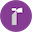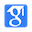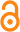# An Improved Analysis of Training Over-parameterized Deep Neural NetworksADVANCES IN NEURAL INFORMATION PROCESSING SYSTEMS 32 (NIPS 2019), pp. 2053-2062, 2019.

Cited by: 43|Bibtex|Views62
EI
Keywords:
Weibo:
We provide an improved analysis of the global convergence of gradient descent for training deep neural networks, which only requires a milder over-parameterization condition than previous work in terms of the training sample size and other problem-dependent parameters

Abstract:

A recent line of research has shown that gradient-based algorithms with random initialization can converge to the global minima of the training loss for over-parameterized (i.e., sufficiently wide) deep neural networks. However, the condition on the width of the neural network to ensure the global convergence is very stringent, which is o...More

Code:

Data:

0
Introduction
• Recent study  has revealed that deep neural networks trained by gradient-based algorithms can fit training data with random labels and achieve zero training error.
• This paper continues the line of research, and improves the over-parameterization condition and the global convergence rate of gradient descent for training deep neural networks.
• Based on Assumptions 3.1 and 3.2, the authors are able to establish the global convergence rates of GD and SGD for training deep ReLU networks.
Highlights
• Recent study  has revealed that deep neural networks trained by gradient-based algorithms can fit training data with random labels and achieve zero training error
• Li and Liang , Du et al  advanced this line of research by proving that under much milder assumptions on the training data, gradient descent can attain a global convergence for training over-parameterized (i.e.,sufficiently wide) two-layer ReLU network with widely used random initialization method 
• This paper continues the line of research, and improves the over-parameterization condition and the global convergence rate of gradient descent for training deep neural networks
• Unlike the gradient descent, which has the same convergence rate and over-parameterization condition for training both deep and two-layer networks in terms of training data size n, we find that the over-parameterization condition of stochastic gradient descent (SGD) can be further improved for training two-layer neural networks
• We studied the global convergence of gradient descent for training overparameterized ReLU networks, and improved the state-of-the-art results
• It is promising that if we can further improve the characterization of "gradient region", as it may provide a tighter gradient lower bound and sharpen the over-parameterization condition. Another interesting future direction is to explore the use of our proof technique to improve the generalization analysis of overparameterized neural networks trained by gradient-based algorithms [1, 6, 4]
Results
• The authors first compare the result with the state-of-the-art proved in Allen-Zhu et al , where they showed that SGD can find a point with ✏-training loss within Oe n7L2 log(1/✏)/(B 2)
• Unlike the gradient descent, which has the same convergence rate and over-parameterization condition for training both deep and two-layer networks in terms of training data size n, the authors find that the over-parameterization condition of SGD can be further improved for training two-layer neural networks.
• This is because for two-layer networks, the training loss enjoys nicer local properties around the initialization, which can be leveraged to improve the convergence of SGD.
• Comparison, Allen-Zhu et al , Zou et al  only leveraged the “gradient region” for one training data point to establish the gradient lower bound, which is shown in Figure 1(b).
• The improved analysis of the trajectory length is motivated by the following observation: at the t-th iteration, the decrease of the training loss after one-step gradient descent is proportional to the gradient norm, i.e., L(W(t)) L(W(t+1)) /
• The authors' proof road map can be organized in three steps: (i) prove that the training loss enjoys good curvature properties within the perturbation region B(W(0), ⌧ ); (ii) show that gradient descent is able to converge to global minima based on such good curvature properties; and (iii) ensure all iterates stay inside the perturbation region until convergence.
• Lemma 4.3 suggests that the training loss L(W) at the initial point does not depend on the number of hidden nodes per layer, i.e., m.
Conclusion
• Similar to the proof in this paper, based on these good properties, the authors can prove that until convergence the neural network weights, including the top layer weights, would not escape from such region.
• The authors studied the global convergence of gradient descent for training overparameterized ReLU networks, and improved the state-of-the-art results.
• Another interesting future direction is to explore the use of the proof technique to improve the generalization analysis of overparameterized neural networks trained by gradient-based algorithms [1, 6, 4].
Summary
• Recent study  has revealed that deep neural networks trained by gradient-based algorithms can fit training data with random labels and achieve zero training error.
• This paper continues the line of research, and improves the over-parameterization condition and the global convergence rate of gradient descent for training deep neural networks.
• Based on Assumptions 3.1 and 3.2, the authors are able to establish the global convergence rates of GD and SGD for training deep ReLU networks.
• The authors first compare the result with the state-of-the-art proved in Allen-Zhu et al , where they showed that SGD can find a point with ✏-training loss within Oe n7L2 log(1/✏)/(B 2)
• Unlike the gradient descent, which has the same convergence rate and over-parameterization condition for training both deep and two-layer networks in terms of training data size n, the authors find that the over-parameterization condition of SGD can be further improved for training two-layer neural networks.
• This is because for two-layer networks, the training loss enjoys nicer local properties around the initialization, which can be leveraged to improve the convergence of SGD.
• Comparison, Allen-Zhu et al , Zou et al  only leveraged the “gradient region” for one training data point to establish the gradient lower bound, which is shown in Figure 1(b).
• The improved analysis of the trajectory length is motivated by the following observation: at the t-th iteration, the decrease of the training loss after one-step gradient descent is proportional to the gradient norm, i.e., L(W(t)) L(W(t+1)) /
• The authors' proof road map can be organized in three steps: (i) prove that the training loss enjoys good curvature properties within the perturbation region B(W(0), ⌧ ); (ii) show that gradient descent is able to converge to global minima based on such good curvature properties; and (iii) ensure all iterates stay inside the perturbation region until convergence.
• Lemma 4.3 suggests that the training loss L(W) at the initial point does not depend on the number of hidden nodes per layer, i.e., m.
• Similar to the proof in this paper, based on these good properties, the authors can prove that until convergence the neural network weights, including the top layer weights, would not escape from such region.
• The authors studied the global convergence of gradient descent for training overparameterized ReLU networks, and improved the state-of-the-art results.
• Another interesting future direction is to explore the use of the proof technique to improve the generalization analysis of overparameterized neural networks trained by gradient-based algorithms [1, 6, 4].
Tables
• Table1: Over-parameterization conditions and iteration complexities of GD for training overparamterized neural
Funding
• This research was sponsored in part by the National Science Foundation CAREER Award IIS-1906169, BIGDATA IIS-1855099, and Salesforce Deep Learning Research Award
• The views and conclusions contained in this paper are those of the authors and should not be interpreted as representing any funding agencies
Reference
• ALLEN-ZHU, Z., LI, Y. and LIANG, Y. (2018). Learning and generalization in overparameterized neural networks, going beyond two layers. arXiv preprint arXiv:1811.04918.• ALLEN-ZHU, Z., LI, Y. and SONG, Z. (2018). A convergence theory for deep learning via over-parameterization. arXiv preprint arXiv:1811.03962.• ALLEN-ZHU, Z., LI, Y. and SONG, Z. (2018). On the convergence rate of training recurrent neural networks. arXiv preprint arXiv:1810.12065.• ARORA, S., DU, S. S., HU, W., LI, Z. and WANG, R. (2019). Fine-grained analysis of optimization and generalization for overparameterized two-layer neural networks. arXiv preprint arXiv:1901.08584.• BRUTZKUS, A. and GLOBERSON, A. (2017). Globally optimal gradient descent for a convnet with gaussian inputs. In Proceedings of the 34th International Conference on Machine LearningVolume 70. JMLR. org.• CAO, Y. and GU, Q. (2019). A generalization theory of gradient descent for learning overparameterized deep relu networks. arXiv preprint arXiv:1902.01384.• CHIZAT, L. and BACH, F. (2018). A note on lazy training in supervised differentiable programming. arXiv preprint arXiv:1812.07956.• DU, S. S. and LEE, J. D. (2018). On the power of over-parametrization in neural networks with quadratic activation. arXiv preprint arXiv:1803.01206.• DU, S. S., LEE, J. D., LI, H., WANG, L. and ZHAI, X. (2018). Gradient descent finds global minima of deep neural networks. arXiv preprint arXiv:1811.03804.• DU, S. S., LEE, J. D. and TIAN, Y. (2017). When is a convolutional filter easy to learn? arXiv preprint arXiv:1709.06129.• DU, S. S., ZHAI, X., POCZOS, B. and SINGH, A. (2018). Gradient descent provably optimizes over-parameterized neural networks. arXiv preprint arXiv:1810.02054.• GAO, W., MAKKUVA, A., OH, S. and VISWANATH, P. (2019). Learning one-hidden-layer neural networks under general input distributions. In The 22nd International Conference on Artificial Intelligence and Statistics.• HE, K., ZHANG, X., REN, S. and SUN, J. (2015). Delving deep into rectifiers: Surpassing human-level performance on imagenet classification. In Proceedings of the IEEE international conference on computer vision.• JACOT, A., GABRIEL, F. and HONGLER, C. (2018). Neural tangent kernel: Convergence and generalization in neural networks. In Advances in neural information processing systems.• LI, Y. and LIANG, Y. (2018). Learning overparameterized neural networks via stochastic gradient descent on structured data. arXiv preprint arXiv:1808.01204.• LI, Y. and YUAN, Y. (2017). Convergence analysis of two-layer neural networks with ReLU activation. arXiv preprint arXiv:1705.09886.• OYMAK, S. and SOLTANOLKOTABI, M. (2019). Towards moderate overparameterization: global convergence guarantees for training shallow neural networks. arXiv preprint arXiv:1902.04674.• TIAN, Y. (2017). An analytical formula of population gradient for two-layered ReLU network and its applications in convergence and critical point analysis. arXiv preprint arXiv:1703.00560.• WU, X., DU, S. S. and WARD, R. (2019). Global convergence of adaptive gradient methods for an over-parameterized neural network. arXiv preprint arXiv:1902.07111.• ZHANG, C., BENGIO, S., HARDT, M., RECHT, B. and VINYALS, O. (2016). Understanding deep learning requires rethinking generalization. arXiv preprint arXiv:1611.03530.• ZHANG, H., YU, D., CHEN, W. and LIU, T.-Y. (2019). Training over-parameterized deep resnet is almost as easy as training a two-layer network. arXiv preprint arXiv:1903.07120.• ZHANG, X., YU, Y., WANG, L. and GU, Q. (2018). Learning one-hidden-layer ReLU networks via gradient descent. arXiv preprint arXiv:1806.07808.• ZHONG, K., SONG, Z., JAIN, P., BARTLETT, P. L. and DHILLON, I. S. (2017). Recovery guarantees for one-hidden-layer neural networks. arXiv preprint arXiv:1706.03175.• ZOU, D., CAO, Y., ZHOU, D. and GU, Q. (2018). Stochastic gradient descent optimizes over-parameterized deep relu networks. arXiv preprint arXiv:1811.08888.Full Text